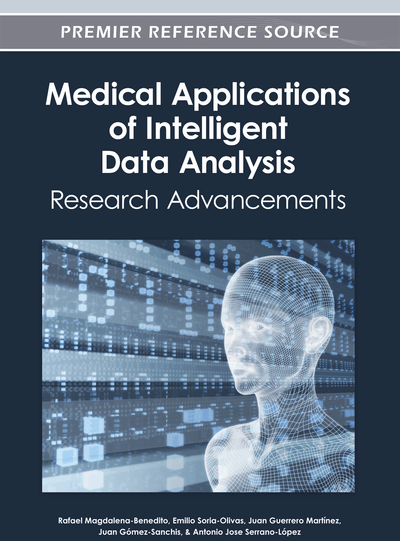# Learning Probabilistic Graphical Models: A Review of Techniques and Applications in Medicine

Juan I. Alonso-Barba (University of Castilla-La Mancha, Spain), Jens D. Nielsen (University of Castilla-La Mancha, Spain), Luis de la Ossa (University of Castilla-La Mancha, Spain) and Jose M. Puerta (University of Castilla-La Mancha, Spain)
DOI: 10.4018/978-1-4666-1803-9.ch015
Available
\$37.50
No Current Special Offers

## Abstract

Probabilistic Graphical Models (PGM) are a class of statistical models that use a graph structure over a set of variables to encode independence relations between those variables. By augmenting the graph by local parameters, a PGM allows for a compact representation of a joint probability distribution over the variables of the graph, which allows for efficient inference algorithms. PGMs are often used for modeling physical and biological systems, and such models are then in turn used to both answer probabilistic queries concerning the variables and to represent certain causal and/or statistical relations in the domain. In this chapter, the authors give an overview of common techniques used for automatic construction of such models from a dataset of observations (usually referred to as learning), and they also review some important applications. The chapter guides the reader to the relevant literature for further study.
Chapter Preview
Top

## Introduction

Probabilistic Graphical Models (PGMs) have been used quite often to model complex problems where there is considerable uncertainty associated. The increased use of these models is due to two main reasons:

• A.

Its graphical representation is very attractive because dependencies between domain variables are represented explicitly, thus it is a powerful tool to describe real phenomena or complex domains.

• B.

Once the probabilistic graphical model is built it is usually relatively easy and efficient to perform the reasoning processes of various types such as predictive reasoning, abductive or diagnostic, backward and forward reasoning, etc.

The problem of learning a PGM from a database of observations has received an enormous amount of attention in the past two decades, and in this chapter we will give an overview of the most common approaches to this problem. We will focus our attention on learning Bayesian Network models, as they comprise the class of models that without doubt has received the most attention in the literature and also those models with the most intuitive interpretation.

Finally, we will give an overview of successful applications in Medical, Bioinformatics and Health fields. Those related to use PGMs as the core representation of the problems, as also by using learning methods to construct such model representation.

Top

## Background: Probabilistic Graphical Models

The focus of this section is how to represent and manage graphical representations of conditional independencies and dependencies in a domain of random variables. We expect the reader to have basic knowledge of conditional and joint probabilities and basic statistics. For a quick review of basic probabilistic and statistical themes, the reader is referred to (DeGroot, 1986).

We will build a graph over a set of random variables by considering each variable as a unique vertex in the graph, and no more vertices are included in the graph. Then, in this graph, the absence of an edge connecting variables A and B represents (marginal or conditional) independence between A and B.

A probabilistic statement of conditional independence is usually denoted aswhere A and B are two random variables and S in the condition or context making A and B independent. Usually S is a subset of the rest of the variables in the domain. In the probabilistic framework we have that:where Pr(A|B,S) is the conditional probability distribution over variable A given the state of variable B and context S. In a graph, an edge connecting two variables A and B can be directedor undirectedwhere undirected edges are also typically referred to as links. Directed edges can represent a causal relation where A is the cause and B the effect of A (e.g. A represents “rain” and B represents “wet grass”). But we can use that representation without any causal interpretation but only the direct relation between the variables. If we have an undirected link connecting the variables we do not suppose in advance any causal or ordered relation between the variables but only a relation “without direction” between the variables.

We can use several types of graphs to represent conditional independencies in a set V of random variables. We can have Directed Acyclic Graph (DAG), where we use only directed edges and without directed cycles. We can use only undirected links yielding an Undirected Graph (UG). It is also possible to use a mixed representation with both kinds of edges, directed an undirected, but again with the constraint of avoiding both directed and semi-directed cycles. Such graphs are usually called Chain Graphs (CG).

In the case of using a DAG, we annotate it with a quantitative part to obtain a (Causal) Bayesian Networks. This model will be the focus of the rest of this chapter.

## Complete Chapter List

Search this Book:
Reset